# An Introduction to Supercapacitors

Published  September 28, 2018   0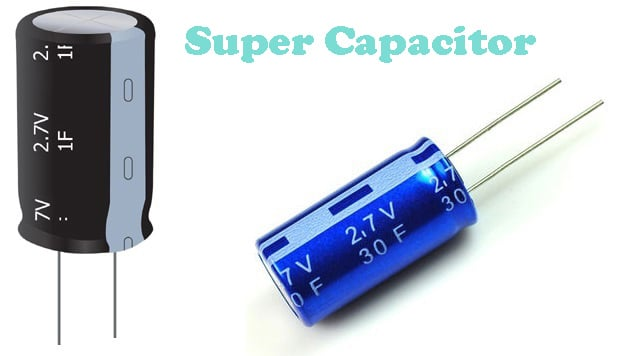The capacitor is a two-terminal passive component, which is widely used in electronics. Almost, every circuit we find in electronics, use one or more capacitors for various usage. Capacitors are the most used electronics component after resistors. They have a special ability to store energy. There are different types of capacitors available in the market, but one which is recently getting popularity and promise a replacement or alternative of batteries in future, are supercapacitors or also known as ultracapacitors. ​A supercapacitor is nothing but a high-capacity capacitor with capacitance values much higher than normal capacitors but lower voltage limits.They can store 10 to 100 times more energy per unit volume or mass than electrolytic capacitors, can receive and deliver charge much faster than batteries, and tolerate more charging-discharging cycles than rechargeable batteries.

Supercapacitors or Ultracapacitors are a new energy storage technology which is developed heavily in modern times. Supercapacitors are providing significant industrial and economic benefits

The capacitance of a capacitor is measured in Farad (F), like .1uF (microfarad), 1mF (millifarad). However, while the lower value capacitors are quite common in electronics, very high-value capacitors are also available, which store energy in much more high density and available in very high capacitance value, ranged in Farad likely.

In the above image, a locally available 2.7V, 1Farad super capacitor image is shown. The voltage rating is much lower but the capacitance of the above capacitor is quite high.

### Benefits of Super-Capacitor or Ultra-Capacitor

The demand of Supercapacitors is rising day by day. The main reason for the rapid development and demand is due to many other benefits of Supercapacitors, few of them are stated below:

• It provides a very good life of approx 1million charge cycles.
• The operating temperature is -50 degrees to 70 degrees almost, which makes it suitable for use in consumer applications.
• A high power density up to 50times, which is achieved by batteries.
• Harmful materials, toxic metals are not the part of the Super Capacitors or Ultracapacitors manufacturing process which makes it certified as the disposable component.
• It is more efficient than batteries.
• Requires no maintenance compared with batteries.

Supercapacitors store energies in its electric field, but in case of batteries, they use chemical compounds to store energies. Also, because of its ability to quick charge and discharge, the Supercapacitors are slowly entering in the battery market. Low internal resistance with very high efficiency, no maintenance cost, higher lifetimes are the main reason for its high demand in the modern power source related market.

### Energies in capacitor

A capacitor store energies in the form of Q = C x V. Q stands for Charge in Coulombs, C for capacitance in Farads and V for voltage in volts. So, if we increase the capacitance the stored energy Q will also increase.

The unit of capacitance is Farad (F) which is named after M. Faraday. Farad is the capacitance unit in respect of coulomb/volt. If we say a capacitor with 1 Farad, then it will create a 1-volt potential difference between its plates depending on the 1-coulomb charge.

1 Farad is a very large value capacitor to use as a general electronic component. In electronics, Generally, microfarad to Pico farad capacitance is used. Microfarad is denoted as uF (1/1,000,000 Farad or 10-6F), nano farad as nF (1/1,000,000,000 or 10-9F) and Pico farad as pF (1/1,000,000,000,000 or10-12F)

If the value becomes much higher, like mF to few Farads (Generally <10F), means the capacitor can holds much more energies between its plates, that capacitor is called as Ultra capacitor or Supercapacitor.

The energies stored in a capacitor is E = ½ CV2 Joules. E is the stored energy in joules, C is the capacitance in Farad and V is the potential difference between the plates.

### Construction of a Supercapacitor

Supercapacitor is an electrochemical device. Interestingly, there are no chemical reactions are responsible to store its electrical energies.​ They have a unique construction, with a large conductive plate or electrode, which are closely situated with a very small surface area. Its construction is the same as an electrolytic capacitor with a liquid or wet electrolyte between its electrodes. You can learn about different types of capacitors here.

Supercapacitor acts as an electrostatic device storing its electrical energy as the electric field between the conductive electrodes.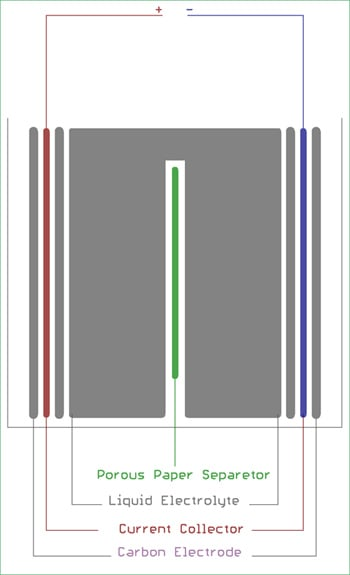The electrodes, Red and blue, are coated double-sided. They generally made of graphite carbon in the form of carbon nanotubes or gels or a special type of conductive activated carbons.

To block the large electron flow between electrodes and passing the positive ion, a porous paper membrane is used. The paper membrane also separates the electrodes. As we can see in the above image, the porous paper membrane is situated in the middle which is green in color. The electrodes and paper separator are impregnated with the liquid electrolyte. The aluminum foil is used as a current collector which establishes the electrical connection.

The separation plate and the area of the plates are responsible for the capacitance value of the capacitor. The relation can be denoted as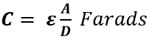Where, Ɛ is the permittivity of the material present between plates

A is the area of the plate

D is the separation between plates

So, in case of supercapacitor, the contact surface is needed to be increased, but there is a limitation. We cannot increase the physical shape or size of the capacitor. To overcome this limitation special type of electrolytes are used to increase the conductivity between plates thus increasing the capacitance.

The supercapacitors also called as double layer capacitor. There is a reason behind it. Very small separation and large surface area using special electrolyte, the surface layer of electrolytic ions form a double layer. It creates two capacitor construction, one at each carbon electrodes and named a double layer capacitor.

These constructions have a drawback. The voltage across the capacitor became very low because of the decomposition voltage of the electrolyte. The voltage is highly dependent on the electrolyte material, the material can limit the electrical energy storing capacity of the capacitor. So, due to the low terminal voltage, an supercapacitor can be connected in series to store electrical charge at a useful voltage level. Due to this, the supecapacitor in series produce higher voltage than usual and in parallel, the capacitance became larger. It can be clearly understood by the below Supercapacitor Array Construction technique.

### Supercapacitor Array construction

To store charge at a useful required voltage, supercapacitors need to be connected in series. And for increasing the capacitance they should be connected in parallel.

Let's see the array construction of the Supercapacitor.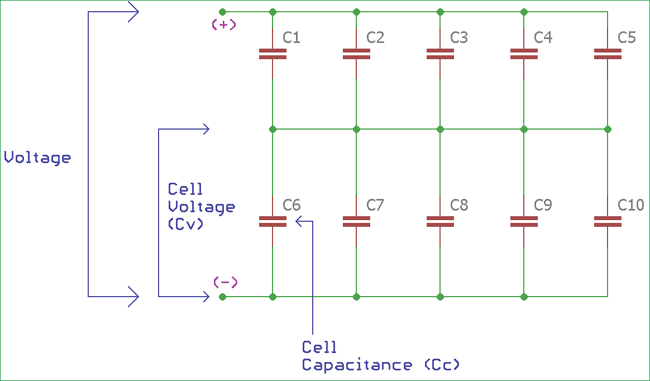In the above image, the cell voltage of a single cell or capacitor is denoted as Cv, whereas the capacitance of a single cell is denoted as Cc. The voltage range of a supercapacitor is from 1V to 3V, the series connections increase the voltage and more capacitors in parallel increase the capacitance.

If we create the array, the voltage in series will be

`Total voltage = Cell Voltage (Cv) x Number of rows`

And the capacitance in parallel will be

`Total capacitance =Cell Capacitance (Cc) x (Number of Column / Number of Row) `

### Example

We need to create a backup storage device, and for that a 2.5F super or supercapacitor is required with the 6V rating.

If we need to create the array using 1F capacitors with the 3V rating, then what will be the array size and capacitors quantities?

```Total voltage = Cell Voltage x Row number
Then, Row number = 6/3
Row number = 2```

Means two capacitor in series will have a 6V potential difference.

Now, the capacitance,

```Total capacitance = Cell capacitance x (Column Number / Row Number)
Then, Coloumn number = (2.5 x 2) / 1 ```

So, we need 2 rows and 5 column.

Let's construct the array,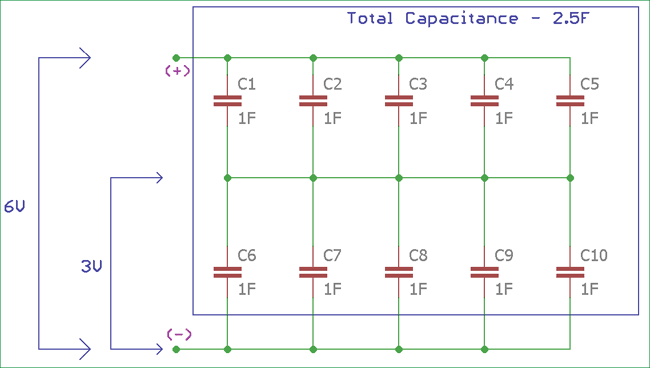The total energy stored in the array is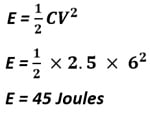Supercapacitors are good in storing energy and where fast charging or discharging is needed. It is widely used as backup devices, where back up power supply or quick discharging is needed. They are further used in Printers, cars and various potable electronics devices.